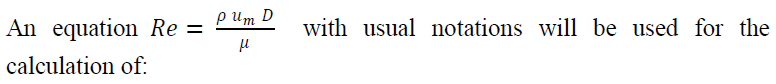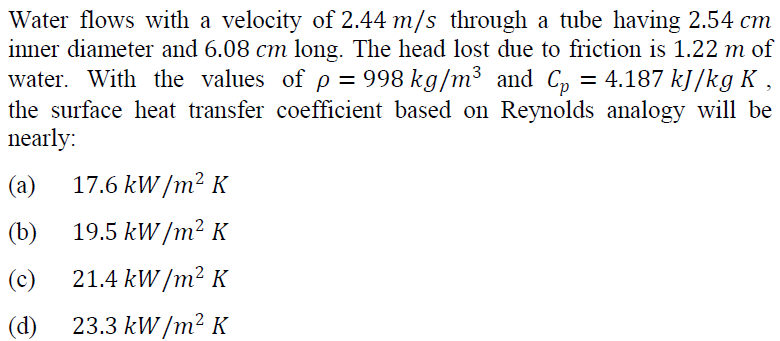61. The ratio of heat transfer rate with fin to the heat transfer rate without fin is:

(a) Fin conductance
(b) Fin efficiency
(c) Fin resistance
(d) Fin effectiveness

62. A 60 W lamp buried in soil (𝑘 =0.83 W/mK) at 0°𝐶 is switched on. The soil temperature at 0.3 m away from the lamp at steady state is:
(a) 18°𝐶
(b) 19°𝐶
(c) 20°𝐶
(d) 21°𝐶

63.(a) Critical Reynolds number for flow over a flat plate
(b) Reynolds number in pipe flow
(c) Flow and heat transfer in a non circular duct, where Dℎ is hydraulic diameter
(d) Reynolds analogy for momentum and heat transfer for turbulent flow

64. The ratio of the buoyancy force to the viscous force acting on the fluid is:
(a) Nusselt number
(b) Reynolds number
(c) Grashof number
(d) Prandtl number

65.66. Consider the following statements with reference to dropwise condensation during heat and mass transfer:
1. Vapour condenses on the surface in the form of drops, and consequently a large part of cooling surface is always bare to vapour for undergoing condensation
2. The rate of heat transfer is many times larger than what is achieved in film condensation
3. It occurs on a non-wettable cooling surface where the liquid condensate drops do not spread

Which of the above statements are correct?
(a) 1 and 2 only
(b) 1 and 3 only
(c) 2 and 3 only
(d) 1, 2 and 3

67. Consider the following statements regarding heat transfer coefficient for laminar flow:
1. When the buoyancy forces are in the same direction as the external forces, they increase the rate of heat transfer
2. When the external and buoyancy forces act in opposite directions, the heat transfer is increased

Which of the above statements is/are correct?
(a) 1 only
(b) 2 only
(c) Both 1 and 2
(d) Neither 1nor 2

68. Consider the following statements regarding velocity distribution in turbulent flow through pipe:
1. No fundamental theory is yet available to determine the velocity distribution
2. Empirical and semi-empirical relations are used to correlate the velocity field in turbulent flow

Which of the above statement is/are correct?
(a) 1 only
(b) 2 only
(c) Both 1 and 2
(d) Neither 1 nor 2

69. A very thin layer at the wall where viscous shear stress dominates is known as:
(a) Buffer sublayer
(b) Viscous sublayer
(c) Turbulent sublayer
(d) Buffer layer